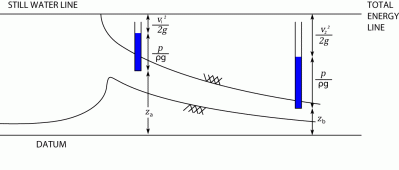I have forgotten

•http://facebook.com/
•https://www.google.com/accounts/o8/id
•https://me.yahoo.com

# Bernoullis Theorem

The background to Bernoulli's Theorem.View other versions (7)

## Overview

Daniel Bernoulli (1700 - 1782) investigated not only mathematics but also such fields as medicine, biology, physiology, mechanics, physics, astronomy, and oceanography.

Key Facts:

Definition
• v is velocity [m/s]
• P is pressure [N/m2]
• g is accretion due to gracity [m/s2]
• z is vertical elevation
• ρ is the density of the liquid

Bernoulli's principle states,
" For a perfect incompressible liquid, flowing in a continuous stream, the total energy of a particle remains the same, while the particle moves from one point to another."
This statement is based on the assumption that there are no losses due to friction in the pipe. Mathematically,
where,
• z = Potential Energy
• = Kinetic Energy
• = Pressure Energy

If a fluid is flowing through a horizontal pipe of varying cross-sectional area, as in Figure 1 for example, the fluid speeds up in constricted areas so that the pressure the fluid exerts is least where the cross section is smallest. This phenomenon is also called the Venturi effect. The Venturi effect has several applications including that associated with generating lift atop an aerofoil, making all forms of flight on aeroplanes, gyroplanes, and helicopters possible.

Note: The Bernoulli's theorem is also the law of conservation of energy, i.e. the sum of all energy in a steady, streamlined, incompressible flow of fluid is always a constant.## Theorem Proof

Consider a perfect incompressible liquid, flowing through a non-uniform pipe as shown in fig.Let us consider two sections AA and BB of the pipe and assume that the pipe is running full and there is a continuity of flow between the two sections. Let,
• = Height of AA above the datum
• = Pressure at AA
• = Velocity of liquid at AA
• = Cross sectional area of the pipe at AA, and
• = Corresponding values at BB

Let the liquid between the two sections AA and BB move to A'A' and B'B' through very small lengths and as shown in fig. This movement of liquid between AA and BB is equivalent to the movement of the liquid between AA and A'A' to BB and B'B', the remaining liquid between A'A' and BB being unaffected. Let, W be the weight of the liquid between AA and A'A'. Since the flow is continuous, therefore
or

Similarly,

We know that the work done by pressure at AA, in moving the liquid to A'A'

Similarly, the work done by pressure at BB, in moving the liquid to B'B'

Note: Minus (-) sign is taken as the direction of is opposite to that of .

Total work done by the pressure

Loss of potential energy =

and again in Kinetic Energy = =

We know that, Loss of potential energy + Work done by pressure = Gain in kinetic energy

which proves Bernoulli's Equation.

Example:
[metric]
##### Example - Bernoulli's Theorem
Problem
The diameter of a pipe changes from 200mm at a section 5m above datum to 50mm at a section 3m above datum. The pressure of water at first section is 500kPa. If the velocity of the flow at the first section is 1m/s, determine the intensity of pressure at the second section.
Workings
Given,
• ,
• ,
• ,
• ,
• and

Let,
• = Velocity of flow at section (2), and
• = Pressure at section (2).

We know that area of the pipe at section (1),

and area of pipe at section (2),

Since the discharge through the pipe is continuous, therefore

Applying Bernoulli's equation for both the ends of the pipe,

Solution
The intensity of pressure at the second section = 392.4 kPa